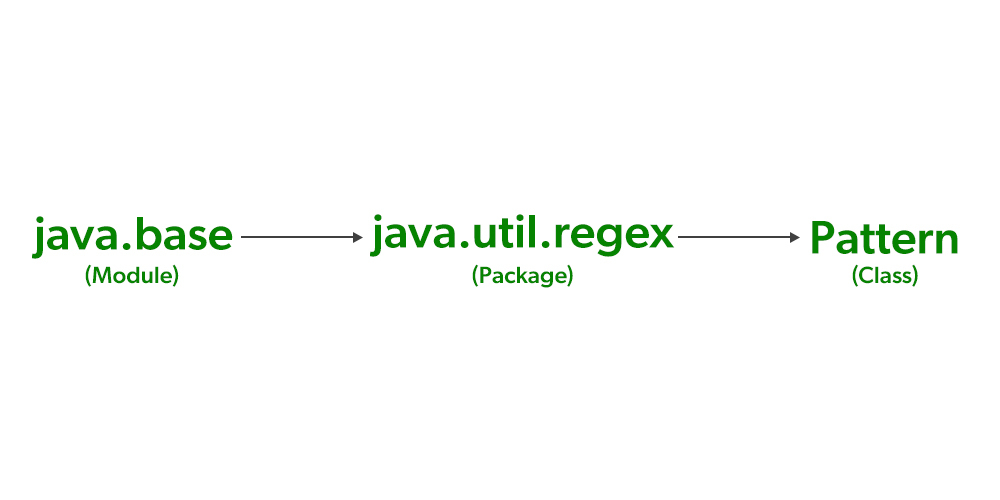Open in App
Not now

# Pattern Class in Java

• Difficulty Level : Easy
• Last Updated : 02 Mar, 2022

The two classes that support Regular Expression (Regex) are as follows, Pattern Class and Matcher Class. Both these classes work together. In simple language, we can think of them as, if we want to define a pattern( regular expression) then we use the Pattern Class and if we want to match that pattern against any other sequence, then we use the Matcher Class.

### Pattern Class

As you can see in the image given below, the Pattern Class belongs to java.util.regex (package), and the package belongs to java.base (module). The pattern class defines no constructor. So, let us see how a pattern is created. The pattern is created using the compile() factory method.

Syntax:

`static Pattern compile(String pattern)`

Here, the pattern in the compile method is a String. This String (pattern ) is then converted into a pattern which can be now used for pattern matching by the Matcher Class. After the pattern is matched, it returns a Pattern object that contains the pattern. The Pattern object created here will now be used to create a Matcher. The Matcher is created by calling the matcher() method defined by Pattern.

Syntax:

`Matcher matcher(CharSequence x)`

Here, x is the character sequence against which the pattern will be matched. Since this is the input you are taking, it is also called the input sequence. CharSequence is an interface that provides read-only access to the set of characters.Example 1:

## Java

 `// Java Program to Demonstrate Pattern Class`   `// Importing required classes` `import` `java.util.regex.*;`   `// Main class` `class` `GFG {`   `    ``// Main driver method` `    ``public` `static` `void` `main(String[] args)` `    ``{` `        ``// Creating a pattern` `        ``Pattern pattern = Pattern.compile(``"GeeksforGeeks"``);`   `        ``// Creating a matcher for the input` `        ``Matcher matcher = pattern.matcher(``"GeeksforGeeks"``);`   `        ``// Checking for a match` `        ``// using matches() method` `        ``boolean` `letsCheck = matcher.matches();`   `        ``// Display message only` `        ``System.out.println(` `            ``" Let us check whether the pattern matches or not:"``);`   `        ``// Condition check whether pattern is matched or not` `        ``if` `(letsCheck)`   `            ``// Matched` `            ``System.out.println(``"Pattern Matched"``);` `        ``else`   `            ``// Not matched` `            ``System.out.println(``"Pattern does not match"``);` `    ``}` `}`

Output

``` Let us check whether the pattern matches or not:
Pattern Matched```

Example 2:

## Java

 `// Java Program to Demonstrate Pattern Class` `// via usage of find() method`   `// Importing required classes` `import` `java.util.regex.*;`   `// Main class` `class` `GFG {`   `    ``// Main driver method` `    ``public` `static` `void` `main(String[] args)` `    ``{` `        ``// Creating a pattern` `        ``Pattern pattern = Pattern.compile(``"GeeksforGeeks"``);`   `        ``// Creating a matcher for the input` `        ``Matcher matcher = pattern.matcher(` `            ``"GFG stands for GeeksforGeeks"``);`   `        ``// Display message only` `        ``System.out.println(` `            ``"Checking for GFG in GeeksforGeeks: "``);`   `        ``// Determining if the input sequence contains the` `        ``// subsequence that matches the pattern` `        ``// using find() method` `        ``if` `(matcher.find())`   `            ``// Found` `            ``System.out.println(``"subsequence GFG found"``);` `        ``else`   `            ``// Not found` `            ``System.out.println(``"subsequence GFG not found"``);` `    ``}` `}`

Output

```Checking for GFG in GeeksforGeeks:
subsequence GFG found```

My Personal Notes arrow_drop_up
Related Articles